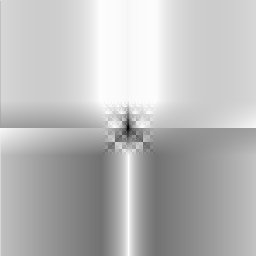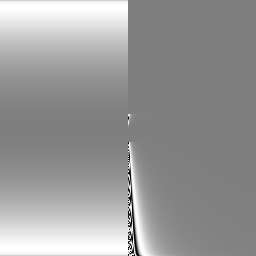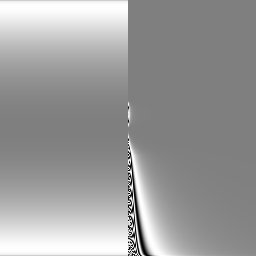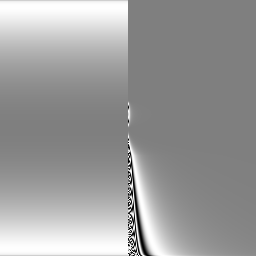# Evolutionary Procedural Modeling with Symbolic Expressions

### a summary by Markus Altmann

Procedural modeling techniques allow the creation of highly complex structures, textures and animations at a low cost [SIMS]. This is performed with a set of parameters - relatively simple input information - and a procedure using these parameters to generate the complexity. A good example for this is a highly detailed mountain terrain generated by fractal subdivision [WATT].

However, these techniques are inevitably specific in their application [WATT]. In addition, the user has to conceive, understand and design the details of the procedure. Another drawback to consider is the difficulty of maintaining sufficient control over the results. This loss of control increases as more options are added to the procedure, to get more variations of the results[SIMS].

With an evolutionary approach, the user still does not have complete control over the detail of the results. However, this approach allows the creation of a large variety of complex entities, resulting from a minimum of user directed input [SIMS]. Moreover, the user himself is not required to understand the creation processes involved and his role is to select the resulting structures to direct the evolution process.

## Definitions

Evolution simulated by a computer involves the same basic concepts as in biology. The genotype is the genetic information (here, a set of procedural parameters, or symbolic expressions), for creating an individual, the phenotype. The process for generating the phenotype from the genotype and the developmental rules (here, procedures), is called expression.
A further process is selection, which determines the fitness of phenotypes, the ability to survive and reproduce. Fitness in simulated evolution can be determined by evaluation of a defined fitness function or, here, by the aesthetic whim of the user [WATT][SIMS].
For evolution to progress, a reproduction of an existing genotype or genotypes should occur, to generate new genotypes. Therefore, there must be variation or mutation in new genotypes with some frequency and then perform the selection on the resulting phenotypes and to get a higher level of fitness [SIMS]. Another way to obtain new genotypes is sexual combination of genetic material from more than one genotype (parents), allowing independently evolved features to be combined into a single individual (child).

## Simulated Evolution within Parameter Space

Here, the genotype is represented by a set of N input parameters (parameter vector [WATT]). Each parameter has an effect in the the expression procedure(s), assembling the phenotype (structure). The possible values of each parameter build up an N-dimensional space, covering the corresponding set of possible structures.

There are two methods for creating structures. One method is the modification of the parameter values by hand for adjusting the structure result. However, predicting the effect of modifications of parameters and combinations of them becomes increasingly difficult as more options are supported by the expression procedure, i.e. as more parameters have to be specified.

The second method allows the selection of a pleasing sample or samples. The samples are generated randomly in the neighborhood of an existing parameter set by random alteration of one or several parameters. This method doesn't require the knowledge of specific effects of each parameter and the parameter space can be explored in incremental arbitrary directions.

### Mutation of Parameter sets

For evolution, the parameter set has to be mutated with some probability during the reproduction. In [SIMS] the parameters of the parent genotype are normalized [0.0, 1.0] and copied to the new child genotype with a certain probability of mutation m (cond.: rand(0.0, 1.0) >= m). In case of mutation (rand(0.0, 1.0) < m), the parameter of the parent is modified by adding a random mutation distance +/-d. Since parameter values are normalized, the mutation distance is proportional to the scale of the value range of the parameter. After mutation, the normalized values are scaled, offset and optionally squared to the appropriate parameter values.

### Mating of Parameter Sets

Mating of two parameter sets, representing different structures with desirable features, enables the combination of these features into a single structure. This reproduction with sexual combination can be accomplished with one of four methods in [SIMS]:

1. Copying gene sequences while switching between parents with some frequency, so adjacent genes are more likely to stick together.

2. Independent copying of each gene from one of the parents with equal probability.

3. Child receives random p% of one parent's genes and (100-p)% from the other. This results into a linear interpolation between parent genotypes for p = 50.

4. Each child gene gets a random value between values of corresponding parent genes (genes independently interpolated).

## Evolution with Symbolic Expressions

Genotypes with fixed parameter sets and fixed expression rules put solid boundaries on the set of possible phenotypes. Using symbolic expressions as genotypes, surpasses these boundaries. Genotypes of symbolic expressions also include procedural information beside the data and are not restricted to a certain structure or size. Therefore, mutation can not only adjust existing expression parts, but also create larger expressions with new parameters.
An expression is generated from random selections in a set of functions, which could be standard mathematical functions/operations, Boolean & image processing operations etc. The necessary function arguments are also randomly chosen and could be scalar, vectors, variables (X, Y pixel coord.) or even another expression, resulting in - desirable - nested expressions. The created expression can then be evaluated by varying the values of the expression variables, corresponding to pixel coordinates (Fig. 1).Fig. 1: Result of the expression:
( -( ( *( ( abs( ( +( X ( ^( 0.000 rand() )) )) )) ( -( ( log( ( abs( ( atan( X )) )) )) ( ^( Y X )) )) )) T )),
X and Y are changed form -1 to 1, T = 1.0

### Mutating symbolic Expressions

The mutation should only slightly modify the expression, because large changes in a genotype usually cause a very different phenotype. Such a phenotype need not to be an improvement, but this is important to extend the expression for more complex structures.

[SIMS] uses a recursive scheme, traversing an expression as a tree structure, for the actual mutation. Each node of the tree can be mutated (see following list), while the frequency of the mutation type depends on the node type: ('->' denotes mutation / becomes)

1. any node -> new random expression, which allows large changes/alternations of genotype/phenotype

2. scalar/vector node -> scalar/vector components, adjusted by addition of random amount

3. function node -> different function (adjustment of arguments necessary)

4. expression -> argument of new random function (necessary arguments generated at random)

5. argument of function -> new node value (inverse of 4.)

6. node -> copy of another node from parent expression in the expression (duplication of sub-expression)

The mutation frequencies are scaled inversely proportional to the length of the parent expression and should be adjusted, so that a lower complexity is slightly more probable than higher one.

### Mating Symbolic Expressions

Here, sub-expressions from different genotypes are mixed into a single individual, and [SIMS] describes two methods to do so:

1. The two parents should be of equal (tree) structure for simultaneous traversal. The similar nodes are copied to the new expression (child), and on differences, one node from the parents is copied with equal probability. The offspring usually will not vary greatly form the parents, and if the parents have different root nodes, no new combination will result.

2. A crossing over technique is used, by replacing a random chosen node of one parent with a random one from the other, allowing even dissimilar expressions to be combined.

### Evolving Volume Texture & Animations

For these purposes additional variables are added to the possible arguments to choose from. The evolution of volume textures involves a Z variable and an adjustment of the function set (e.g. 3D solid noise generation) to evaluate expressions in 3D space.

Evolution of animations can also be done by including a Time variable into the expression, so images are produced for smooth changes of Time (Fig 2).Fig 2: Lifting the curtain of complexity (just a bit - left to right/top to bottom). Expression used:
( sin( ( sqr( ( *( ( asin( ( atan( ( tan( Y )) )) )) ( /( ( &( T Y )) X )) )) )) ))
X & Y like in Fig. 1, T = 0.01 to 0.31 in steps of 0.1

There are four more methods for animation in [SIMS]:

1. Genetic cross dissolve between two expressions of similar structure, which is performed by interpolating the results where the expressions differ. These results are first calculated & dissolved, and then used by the remaining expression parts to generate a smooth transition between the two expressions.

2. Instead of a Time argument, an Image argument can be integrated into an expression. This allows complex manipulations of images, whose values are used by the expression evaluation. The values of Image correspond to frames of an existing animation.

3. When using pixel coordinates (X, Y) to determine the pixel color, altering the mapping of X & Y, e.g. via zooming, 3D perspective transformation, etc., before expression evaluation, animates an image.

4. Smooth interpolation of parameters in expression to new values.

Finally, all above mentioned methods for animation can be combined.

## Conclusion

Artificial evolution is a powerful tool for the procedural generation of structures, texture & motion. When using genotypes with symbolic expressions, there are no limits in complexity and no understanding is required for creating & exploring the complexity. The interactive work of user & computer can produce results, neither could do alone. In order to allow interactive work, fast generation of samples to choose from is necessary. Finally, all the results can be saved in a concise form by the genotype itself, i.e. the formulation of the symbolic expression.

References
[SIMS]
Karl Sims,
"Artificial Evolution for Computer Graphics",
July 1991, Computer Graphics, Vol. 25, No. 4, pp. 319 - 328

[WATT]
Alan Watt, Mark Watt,